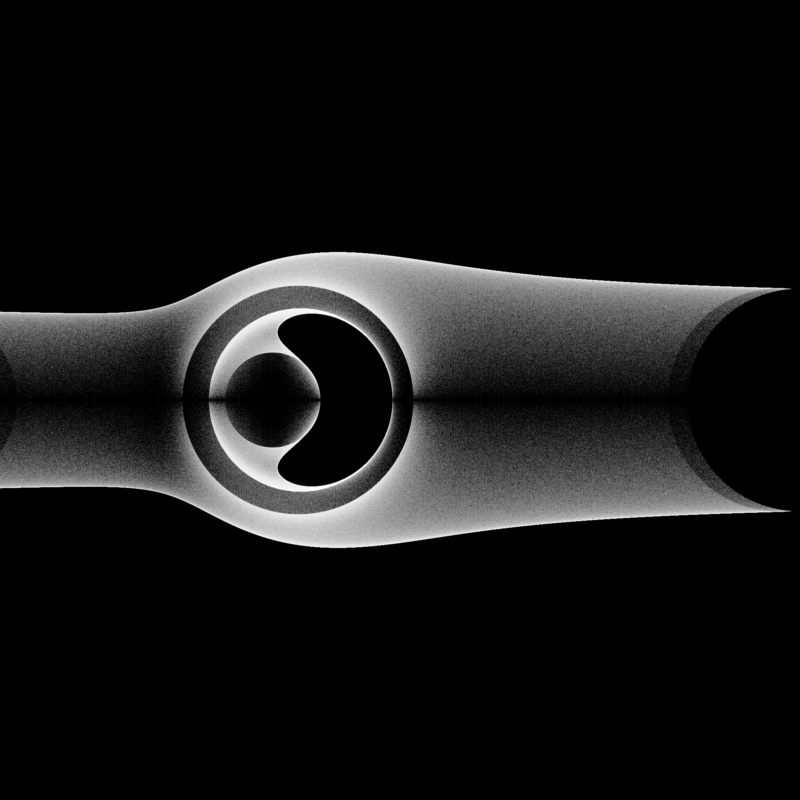# Bohemian MatricesEigenvalues of the matrix $$\begin{bmatrix} A & 1 & -1\\ -1 & B & 0\\ 1 & -1 & -1 \end{bmatrix}$$ where $A$ and $B$ are independent continuous uniform random variables on $(-5, 4)$. This image represents a sample of 5 million matrices. Note that this class of matrices is not Bohemian as it is sampled from a continuous distribution. Viewed on [-4-4i, 4+4i]. Real eigenvalues have been omitted from this plot.